# MCAT Physical : Endergonic and Exergonic Reactions

## Example Questions

### Example Question #1 : Endergonic And Exergonic Reactions

Boiling point is the temperature a liquid needs to achieve in order to begin its transformation into a gaseous state. Campers and hikers who prepare food during their trips have to account for differences in atmospheric pressure as they ascend in elevation. During the ascent, the decrease in atmospheric pressure changes the temperature at which water boils.

Further complicating the matter is the observation that addition of a solute to a pure liquid also changes the boiling point. Raoult’s Law can be used to understand the changes in boiling point if a non-volatile solute is present, as expressed here.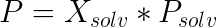In this law,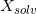is the mole fraction of the solvent,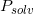is the vapor pressure of the pure solvent, andis the vapor pressure of the solution. When this vapor pressure is equal to the local atmospheric pressure, the solution boils.

A scientist is studying solution chemistry to better understand vapor pressure. He finds that, for one solution he creates, the beaker is cool to the touch after the solute is fully dissolved. Which of the following is true of this solution? (Note: The beaker, solute, and solvent are the system, the remainder of the universe is the surroundings)

It forms spontaneously only at low temperatures

It forms spontaneously only if dissolution decreases entropy of the system

It always forms spontaneously

It forms spontaneously only at high temperatures

It never forms spontaneously

It forms spontaneously only at high temperatures

Explanation:

The act of dissolving a solute in a solvent is a local increase in entropy, converting a single molecule to multiple ions. The absorption of heat from the surroundings (cool beaker) indicates that this is an endothermic dissolution. We can look at the equation for Gibbs free energy to evaluate the possible answers.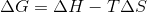In order to be spontaneous, the reaction must have a negative Gibbs free energy. To accomplish this, a reaction may have a negative enthalpy (exothermic) and positive entropy, however we know that our reaction has a positive enthalpy (endothermic) and positive entropy. A reaction will be spontaneous if it has a positive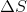and a positive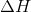only when temperature is high.

### Example Question #2 : Endergonic And Exergonic Reactions

A scientist is studying a reaction, and places the reactants in a beaker at room temperature. The reaction progresses, and she analyzes the products via NMR. Based on the NMR readout, she determines the reaction proceeds as follows: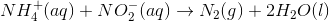In an attempt to better understand the reaction process, she varies the concentrations of the reactants and studies how the rate of the reaction changes. The table below shows the reaction concentrations as she makes modifications in three experimental trials.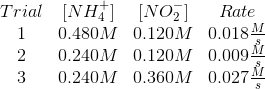Assume that the forward reaction in the passage is exothermic, and has avalue of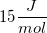.  Which of the following is true?

The spontaneity of the reaction cannot be predicted

The reaction is never spontaneous

The reaction is always spontaneous

The reaction is spontaneous only at low temperatures

The reaction is spontaneous only at high temperatures

The reaction is always spontaneous

Explanation:

The question specifies that this reaction is exothermic, and that it has a positive local increase in entropy as it progresses. This means that the change in enthaply is negative and the change in entropy is positive.

For a reaction to be spontaneous, Gibbs free energy must be negative.If enthalpy is negative and entropy is positive, the temperature is irrelevant and Gibbs free energy will always be negative.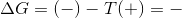The reaction is spontaneous at any temperature.

### Example Question #3 : Endergonic And Exergonic Reactions

For “Reaction A,”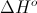is +300kJ and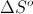is -98J. The reaction will be spontaneous under which of the following conditions?

High temperature

The reaction cannot be spontaneous

Low temperature

Moderate temperature and high pressure

The reaction is always spontaneous

The reaction cannot be spontaneous

Explanation:

The spontaneity of a reaction is determined by the equation for Gibbs free energy.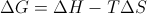Here, H is a positive number and S is negative, meaning that G will always be a positive value. T will be given in Kelvin, and cannot be negative. Reactions with positive Gibbs free energy values are never spontaneous.

### Example Question #4 : Endergonic And Exergonic Reactions

The equation for the change in Gibbs free energy is given below.ΔH = change in enthalpy

ΔS = change in entropy

Which of the following scenarios guarantees a nonspontaneous reaction?

When ΔH is positive and ΔS is negative

When ΔH is positive and ΔS is positive

When ΔH is negative and ΔS is negative

When ΔH is negative and ΔS is positive

When ΔH is positive and ΔS is negative

Explanation:

A positive value for ΔG (Gibbs free energy) will guarantee a nonspontaneous reaction. When ΔH (enthalpy) is postive and ΔS (entropy) is negative, the change in Gibbs free energy must be positive and, therefore, nonspontaneous.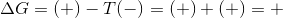Because T (temperature) will always have a positive value, a negative entropy and positive enthalpy will always result in a positive Gibbs free energy.

### Example Question #5 : Endergonic And Exergonic Reactions

The equation for the change in Gibbs free energy is given below.ΔH = change in enthalpy

ΔS = change in entropy

In which of the scenarios will the reaction be spontaneous?

When ΔH is negative and ΔS is postive

When both ΔH and ΔS are negative and T is low

All of these scenarios would result in spontaneous reactions

When both ΔH and ΔS are postive and T is high

All of these scenarios would result in spontaneous reactions

Explanation:

All of the following scenarios would lead to spontaneous reaction, since each scenario would result in a negative Gibbs free energy (-ΔG).Negative enthalpy, positive entropy: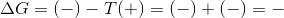Positive enthalpy and entropy with high temperature: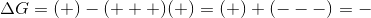Negative enthalpy and entropy with low temperature: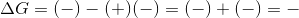### Example Question #6 : Endergonic And Exergonic Reactions

Which of the following scenarios describes a reaction in equilibrium?

When the enthalpy is equal to the entropy

When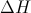is equal to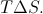When the forward rate is 0M/s

When Gibbs free energy is negative

Whenis equal toAccording to the Gibbs free energy equation, a system is at equilibrium when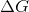is equal to 0.
Since, a system is in equilibrium when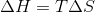. A negative Gibbs free energy means that the reaction will be spontaneous. At equilibrium, the forward reaction rate equals the reverse reaction rate, though the net rate is zero.